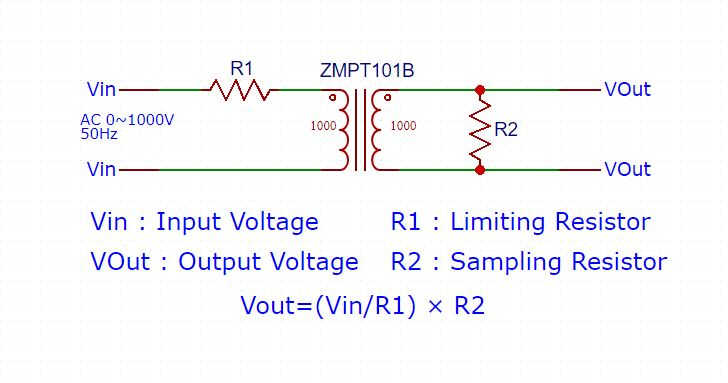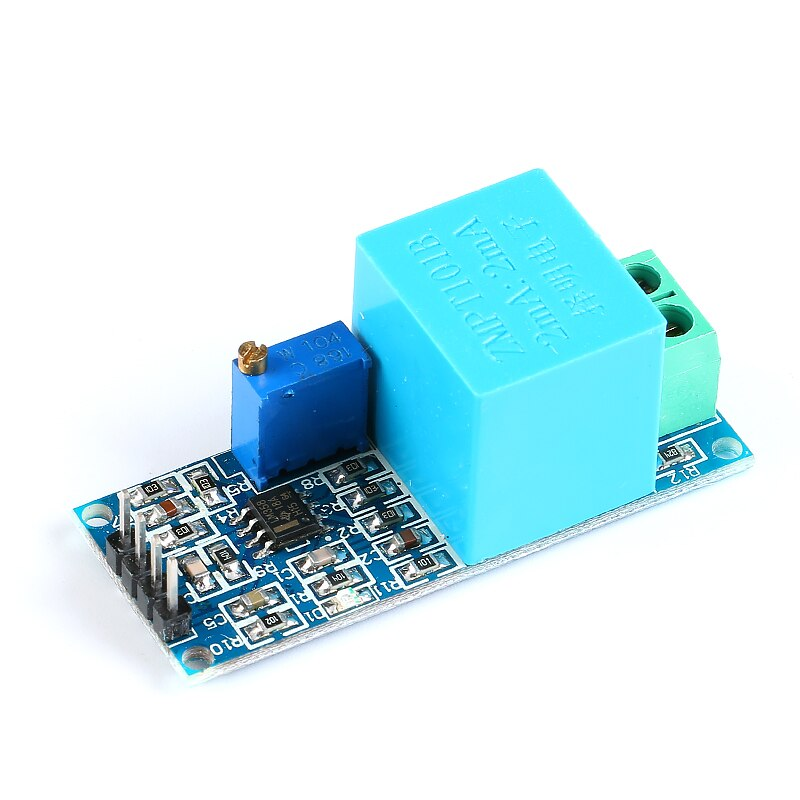ZMPT101B AC Voltage Sensor is the best for the purpose of the DIY project, where we need to measure the accurate AC voltage with voltage transformer. This is an ideal choice to measure the AC voltage using Arduino/ESP8266/Raspberry Pi like an opensource platform. In many electrical projects, engineer directly deals with measurements with few basic requirements like

• High galvanic isolation
• Wide Range
• High accuracy
• Good Consistency

ZMPT 101B is a high precision voltage Transformer. This module makes it easy to monitor AC mains voltage upto 1000 volts. if you want smaller version of this module check ZMPT107.

## Applications of Small Size Voltage transformer

• Metering (electrical energy meters)
• AC Voltage measurment
• Ground fault detection
• Household electrical equipment
• Industrial apparatuses
• Electrical testing equipment and relay protection

## Features of ZMPT 101B Micro Precision Voltage Transformers

• low price
• small size (49.5mm x 19.4mm) and lightweight
• Easy PCB mounting
• Good consistency
• widely acclaimed
Specifications Discriptions
Rated input current 2mA
Rated output current 2mA
Linear range 0~1000V 0~10mA
Isolation withstand voltage 4000V
Turns Ratio 1000:1000
Measurement accuracy Class 0.2
Linearity 0.1%
Rated Burdon <=200Ohm
Operating Frequency 50-50Hz
DC coil Resistance 110 @ 20 deg Cel

## Know More About Voltage Transformer

ZMPT101B is a high precision voltage Transformer. This module makes it easy to monitor AC mains voltage upto 1000 volts. A tiny little thing the size of a bouillon cube. Holds up to 4kV per breakdown voltage, the ratio of turns is 1: 1, but this is a current transformer of 2mA: 2mA. That is, we feed it a current and remove the current. The input current is simply set by the resistor in series R1, and a sampling resistor R2 is used in parallel to obtain the output voltage.

## ZMPT101B schematic Diagram## Now we get 5 volts, but here an ambush. This voltage is vary from -5 to +5. And we need measurements of 0 … 5 volts. What to do? Straighten.

See in next section.

## ZMPT 101B Transformer Calculation

[ratemypost]

R1 is chosen so that the current through the winding does not exceed 2mA, it holds a maximum of 10mA, but after 2mA linearity is lost and the output will be clear that.

### Step 1: Determination of maximum output rms voltage

VOutmax is decided by the ADC peak voltage in the sampling loop of Microcontroller.

VOutmax=(Peak Voltage)/(√2)

For example
As for ± 5V ADC，the maximum rms voltage of the transformer:

VOutmax=(Peak Voltage)/(√2)=5V/(√2)=3.53V

VOutmax=(Peak Voltage)/(2√2)

For example
As for 0-3.3V ADC，the maximum rms voltage of the transformer:

VOutmax=(Peak Voltage)/(2√2)=3.3V/(2√2)=1.16V

### Step 2: Determination of input current-limiting resistor R1

Current-limiting resistor

R1=Vin/I

Where
Vin：Rated input voltage
I ： Rated operating current （ when Coil resistance is compared with current-limiting resistor R1, it can be ignored.）

ZMPT101B usually working at rated current: 1~2mA.
When Rated input voltage ≤ 100V ，Usually choosing the operating current I=2mA; When Rated input voltage ≥ 220V，To reducing the resistor power， usually choosing the operating current 1 mA ≤ I ≤ 2 mA.
for example: V=100V，I=2 mA，

R1=Vin/I=100/0.002=50kΩ

for example: V=220V，I=1.1 mA，

R1=Vin/I=220/0.0011=200kΩ

To improve reliability, the current-limiting resistor selected usually is greater than its 4 times the rated power, and generally, use a high temperature coefficient metal film resistor.

### Step 3: Determination of the sampling resistor R2

R2=VOutmax/I=VOutmax/Vin×R1 Ω

for example: Voutmax =3.53V，Vin =100V

R2=VOutmax/I=VOutmax/Vin×R1=3.53/100×50 kΩ=1.765kΩ

Above formula is also suitable for the two ways of active and passive output.
When selecting the sampling resistor, Resistor should not exceed

R2=VOutmax/I=VOutmax/Vin×R1 Ω

## Interface ZMPT 101 B Module to Arduino

For Diy Electronic measuring AC Output Voltage using Arduino is easy now.

ZMPT101B is a Single Phase Voltage Transformer Module which allows you to directly measure 0-250V AC sine wave using Arduino.

Description:
Single-phase AC active output voltage mutual inductance module equipped with ZMPT101B series of a high-precision voltage transformer and high-precision op-amp current, easy to 250v within the AC power signal acquisition.## Feature of ZMPT101B Module

• Voltage Transformer: Onboard Precision Micro Voltage Transformer
• Operational amplifier circuit: high-precision on-board amplifier circuit, the signal to do the exact sampling and appropriate compensation.
• Input/Output: the module can measure AC voltage within 250V, the corresponding output voltage amplitude can be adjusted by using a potentiometer( Multi-turn trim pot).
• Output signal: the output signal for the sine wave, the waveform of the median (DC component(offset of 2.5V at 5V input supply)
• Supply voltage: 5V
• Operating temperature: 40ºC ~ + 70ºC